# OAE Middle Grades Math: Circular Arcs & Measurement Chapter Exam

Exam Instructions:

Choose your answers to the questions and click 'Next' to see the next set of questions. You can skip questions if you would like and come back to them later with the yellow "Go To First Skipped Question" button. When you have completed the practice exam, a green submit button will appear. Click it to see your results. Good luck!

### Page 1

#### Question 1 1. What is the area of the shaded area, rounded to the nearest whole number?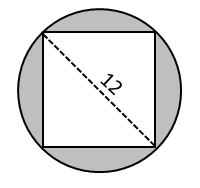#### Question 2 2. If the area of the square is 64, what is the area of the circle, rounded to the nearest whole number?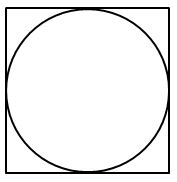#### Question 4 4. In the picture below, if arc BD is 168 degrees and arc BC is 70 degrees, what is the measure of angle BAD?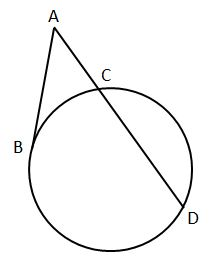### Page 2

#### Question 7 7. If arc AB is 72 degrees, what is the measure of angle ACB?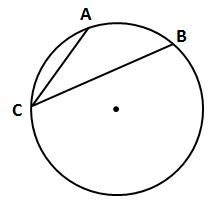#### Question 9 9. If arc BC is 84 degrees, what is the measure of angle ACB?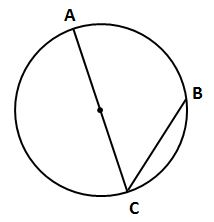#### Question 10 10. Which line is a tangent to the pictured circle?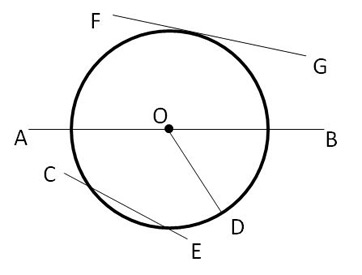### Page 3

#### Question 12 12. In the picture below, if major arc BC is 258 degrees and minor arc BC is 102 degrees, what is the measure of angle BAC?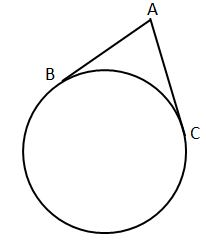#### Question 14 14. If AB and CB are tangent segments, what is the length of CB?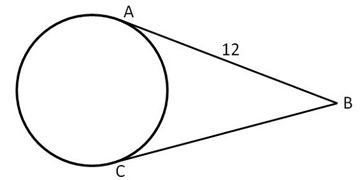### Page 4

#### Question 16 16. In the picture below, if arc CE is 172 degrees and arc BD is 80 degrees, what is the measure of angle CAE?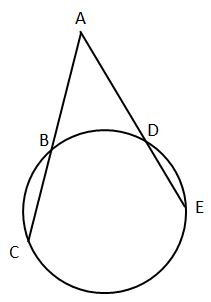#### Question 18 18. In the picture below, if arc AB is 82 degrees, what is the measure of angle BAC?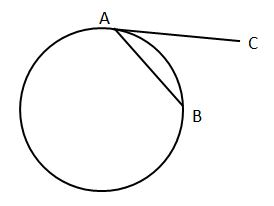#### Question 20 20. In the picture below, if AC is 14 and AB is 10, what is the length of AD?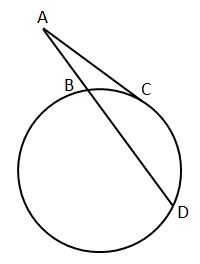### Page 5

#### Question 21 21. In the picture below, if AB is 12, AC is 24 and AD is 10, what is the length of AE?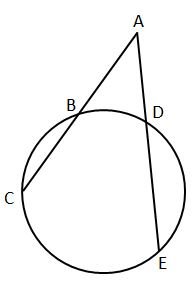### Page 6

#### Question 29 29. If arc AC is 106 degrees and arc BC is 82 degrees, what is the measure of angle ACB?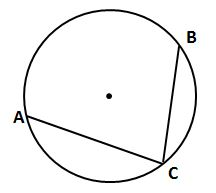#### OAE Middle Grades Math: Circular Arcs & Measurement Chapter Exam Instructions

Choose your answers to the questions and click 'Next' to see the next set of questions. You can skip questions if you would like and come back to them later with the yellow "Go To First Skipped Question" button. When you have completed the practice exam, a green submit button will appear. Click it to see your results. Good luck!

Support